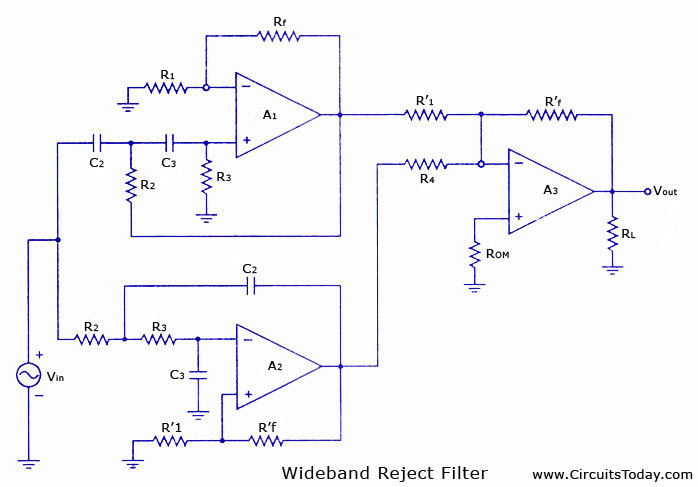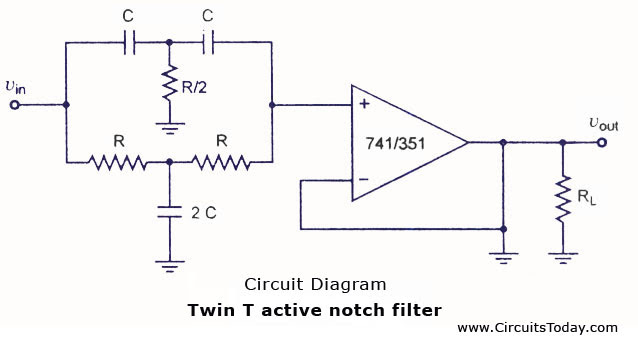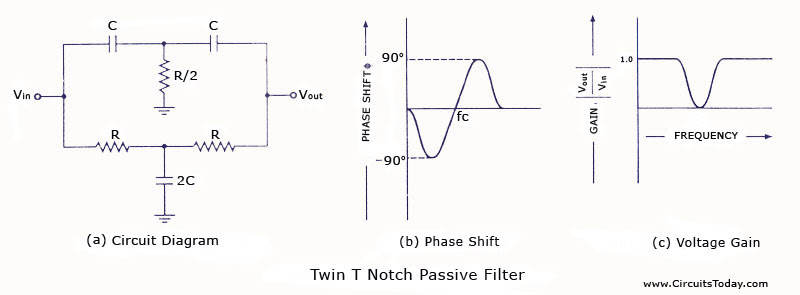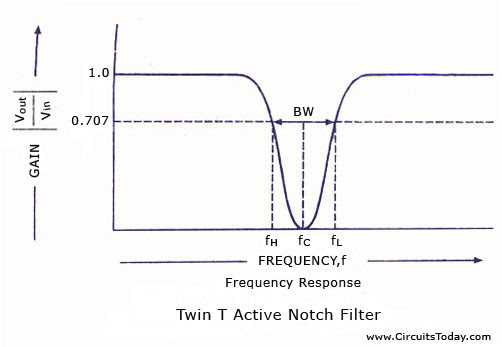## Audio

[Audio][slideshow]

The bandpass fil­ter passes one set of frequen­cies while reject­ing all others. The band-stop filter does just the opposite. It rejects a band of frequencies, while passing all others. This is also called a band-reject or band-elimination filter. Like band­pass filters, band-stop filters

may also be classified as (i) wide-band and (ii) narrow band reject filters.

The narrow band reject filter is also called a notch filter. Because of its higher Q, which exceeds 10, the bandwidth of the narrow band reject filter is much smaller than that of a wide band reject filter.

### Wide Band-Stop (or Reject) Filter.Wide band stop filter

A wide band-stop filter using a low-pass filter, a high-pass filter and a summing amplifier is shown in figure. For a proper band reject response, the low cut-off frequency fL of high-pass filter must be larger than the high cut-off frequency fH of the low-pass filter. In addition, the passband gain of both the high-pass and low-pass sections must be equal.

### Narrow Band-Stop Filter.This is also called a notch filter. It is commonly used for attenuation of a single frequency such as 60 Hz power line frequency hum. The most widely used notch filter is the twin-T network illustrated in fig. (a). This is a passive filter composed of two T-shaped networks. One T-network is made up of two resistors and a capacitor, while the other is made of two capacitors and a resistor.One drawback of above notch filter (pas­sive twin-T network) is that it has relatively low figure of merit Q. However, Q of the network can be increased significantly if it is used with the voltage follower, as illustrated in fig. (a). Here the output of the volt­age follower is supplied back to the junction of R/2 and 2 C. The frequency response of the active notch filter is shown in fig (b).Notch filters are most commonly used in communications and biomedical instruments for eliminating the undesired frequencies.

A mathematical analysis of this circuit shows that it acts as a lead-lag circuit with a phase angle, shown in fig. (b). Again, there is a frequency fc at which the phase shift is equal to 0°. In fig. (c), the voltage gain is equal to 1 at low and high frequencies. In between, there is a frequency fc at which voltage gain drops to zero. Thus such a filter notches out, or blocks frequencies near fc. The frequency at which maximum attenuation occurs is called the notch-out frequency given by

fn = Fc = 2∏RC

Notice that two upper capacitors are C while the capacitor in the centre of the network is 2 C. Similarly, the two lower resistors are R but the resistor in the centre of the network is 1/2 R. This relationship must always be maintained.

[Lights][grids]